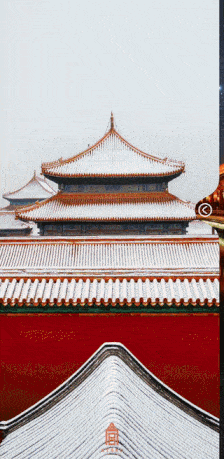# 带你实现女朋友欲罢不能的网易云音乐宇宙尘埃特效

17,881

## 前言

0202年了，Android开发大都应该是老油条了把。如果你自定义View还是掌握得不够熟练的话，那可就说不过去了哦。自定义View可以说是Android开发中，无论是初级，中级还是高级都必须掌握的一个点。ps：为了能更快加载出来，gif是压缩了又压缩，大家可以脑部清晰度。

ps2：小伙伴如果有好的gif压缩网站可以推荐一波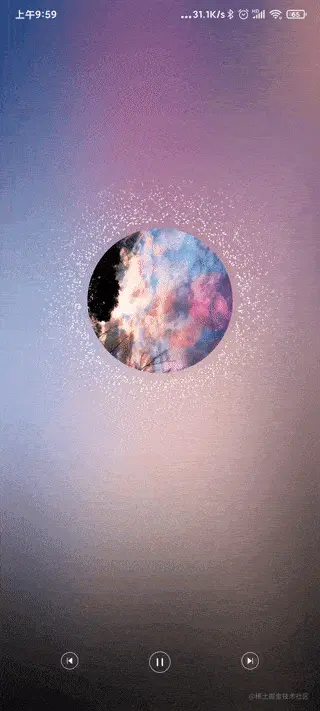## 加载图片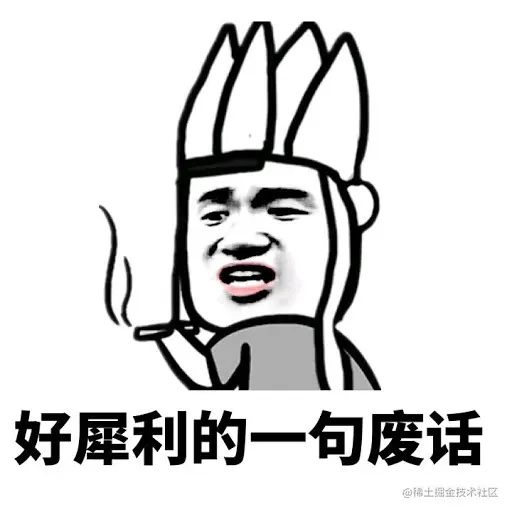<?xml version="1.0" encoding="utf-8"?>
<RelativeLayout xmlns:android="http://schemas.android.com/apk/res/android"
xmlns:app="http://schemas.android.com/apk/res-auto"
xmlns:tools="http://schemas.android.com/tools"
android:id="@+id/rootLayout"
android:layout_width="match_parent"
android:layout_height="match_parent"
android:fitsSystemWindows="true">

<ImageView
android:id="@+id/music_avatar"
android:layout_centerInParent="true"
android:layout_width="178dp"
android:layout_height="178dp"/>

</RelativeLayout>


class DemoActivity : AppCompatActivity() {

private lateinit var demoBinding: ActivityDemoBinding

override fun onCreate(savedInstanceState: Bundle?) {
super.onCreate(savedInstanceState)
demoBinding = ActivityDemoBinding.inflate(layoutInflater)
setContentView(demoBinding.root)

lifecycleScope.launch(Dispatchers.Main) {
}
}
withContext(Dispatchers.IO) {
Glide.with(this@DemoActivity)
.circleCrop()
.into(object : ImageViewTarget<Drawable>(demoBinding.musicAvatar) {
override fun setResource(resource: Drawable?) {
demoBinding.musicAvatar.setImageDrawable(resource)
}
})
}
}
}


## 旋转图片

lateinit var rotateAnimator: ObjectAnimator

override fun onCreate(savedInstanceState: Bundle?) {
...
setContentView(demoBinding.root)
rotateAnimator = ObjectAnimator.ofFloat(demoBinding.musicAvatar, View.ROTATION, 0f, 360f)
rotateAnimator.duration = 6000
rotateAnimator.repeatCount = -1
rotateAnimator.interpolator = LinearInterpolator()
lifecycleScope.launch(Dispatchers.Main) {
//添加点击事件，并且启动动画
demoBinding.musicAvatar.setOnClickListener {
rotateAnimator.start()
}
}
}


## 粒子动画### 粒子动画分析

• 圆形生产粒子
• 粒子速度不同，也就是随机。
• 粒子透明度不断降低，直到最后消散。
• 粒子沿着到圆心的反方向扩散。

### 生产粒子

#### 定义粒子

class Particle(
var x:Float,//X坐标
var y:Float,//Y坐标
var speed:Float,//速度
var alpha: Int//透明度
)


#### 定义自定义view

class DimpleView(context: Context?, attrs: AttributeSet?) : View(context, attrs) {
//定义一个粒子的集合
private var particleList = mutableListOf<Particle>()
//定义画笔
var paint = Paint()
}


override fun onDraw(canvas: Canvas) {
super.onDraw(canvas)
paint.color = Color.WHITE
paint.isAntiAlias = true
var particle=Particle(0f,0f,2f,2f,100)
}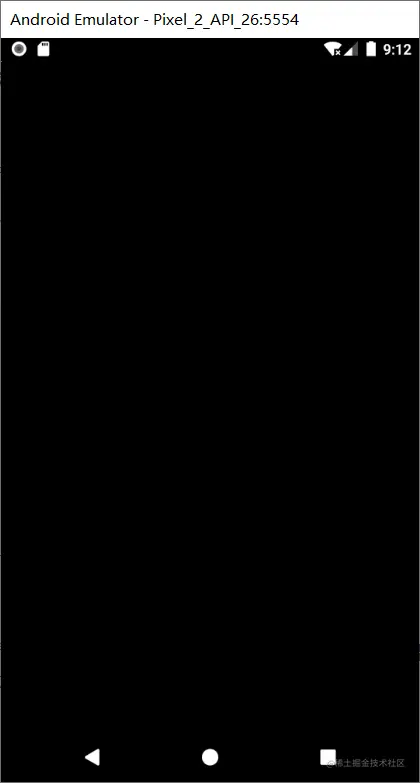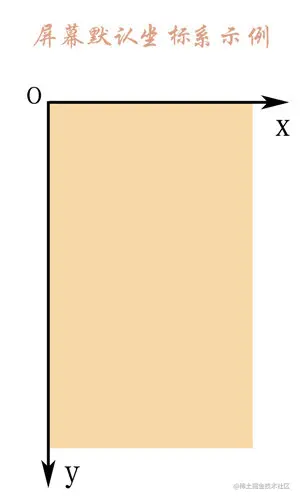override fun onSizeChanged(w: Int, h: Int, oldw: Int, oldh: Int) {
super.onSizeChanged(w, h, oldw, oldh)
centerX= (w/2).toFloat()
centerY= (h/2).toFloat()
}


override fun onDraw(canvas: Canvas) {
...
var particle=Particle(centerX,centerY,2f,2f,100)
}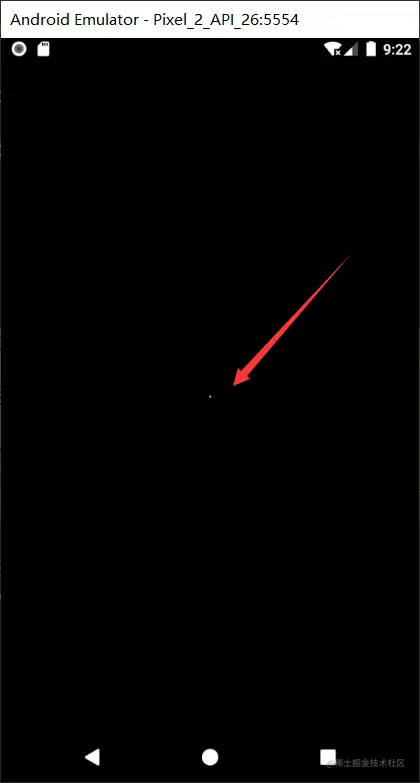##### 添加多个粒子

override fun onDraw(canvas: Canvas) {
super.onDraw(canvas)
paint.color = Color.WHITE
paint.isAntiAlias = true
for (i in 0..50){
var random= Random()
var nextX=random.nextInt((centerX*2).toInt())
var particle=Particle(nextX.toFloat(),centerY,2f,2f,100)
}

}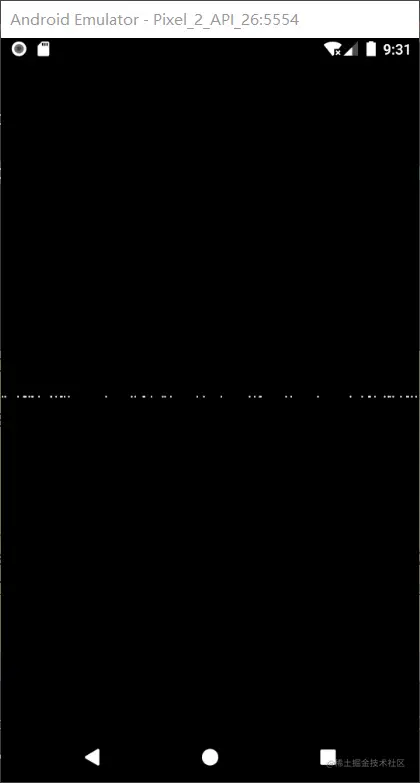override fun onDraw(canvas: Canvas) {
super.onDraw(canvas)
paint.color = Color.WHITE
paint.isAntiAlias = true
var time= measureTimeMillis {
for (i in 0..50){
var random= Random()
var nextX=random.nextInt((centerX*2).toInt())
var particle=Particle(nextX.toFloat(),centerY,2f,2f,100)
}
}
Log.i("dimple","绘制时间$time ms") }  结果如下：50个粒子的绘制时间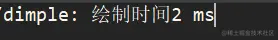5000个粒子的绘制时间：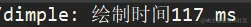可以看到，明显超了16ms。所以我们需要优化，怎么优化？很简单，就是不在ondraw方法中创建对象就好了，那我们选择在哪里呢？ 构造方法可以吗？好像不可以呢，这个时候还没办法获得屏幕宽高，嘿嘿嘿，onSizeChanged方法就决定是你了！ ##### 粒子添加到集合中 override fun onSizeChanged(w: Int, h: Int, oldw: Int, oldh: Int) { super.onSizeChanged(w, h, oldw, oldh) centerX= (w/2).toFloat() centerY= (h/2).toFloat() val random= Random() var nextX=0 for (i in 0..5000){ nextX=random.nextInt((centerX*2).toInt()) particleList.add(Particle(nextX.toFloat(),centerY,2f,2f,100)) } }  我们再来看看onDraw方法中绘制时间是多少： override fun onDraw(canvas: Canvas) { super.onDraw(canvas) paint.color = Color.WHITE paint.isAntiAlias = true var time= measureTimeMillis { particleList.forEach { canvas.drawCircle(it.x,it.y,it.radius,paint) } } Log.i("dimple","绘制时间$time ms")
}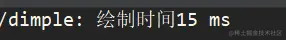emmmm,好像是低于16ms了，可是这也太危险了吧，你这分分钟就超过了16ms啊。

### 定义动画

private var animator = ValueAnimator.ofFloat(0f, 1f)
init {
animator.duration = 2000
animator.repeatCount = -1
animator.interpolator = LinearInterpolator()
updateParticle(it.animatedValue as Float)
invalidate()//重绘界面
}
}


updateParticle方法应该去做什么事情呢？我们来开动小脑筋想想。

#### 更新粒子位置

private fun updateParticle(value: Float) {
particleList.forEach {
it.y += it.speed
}
}
override fun onSizeChanged(w: Int, h: Int, oldw: Int, oldh: Int) {
...
animator.start()//别忘了启动动画
}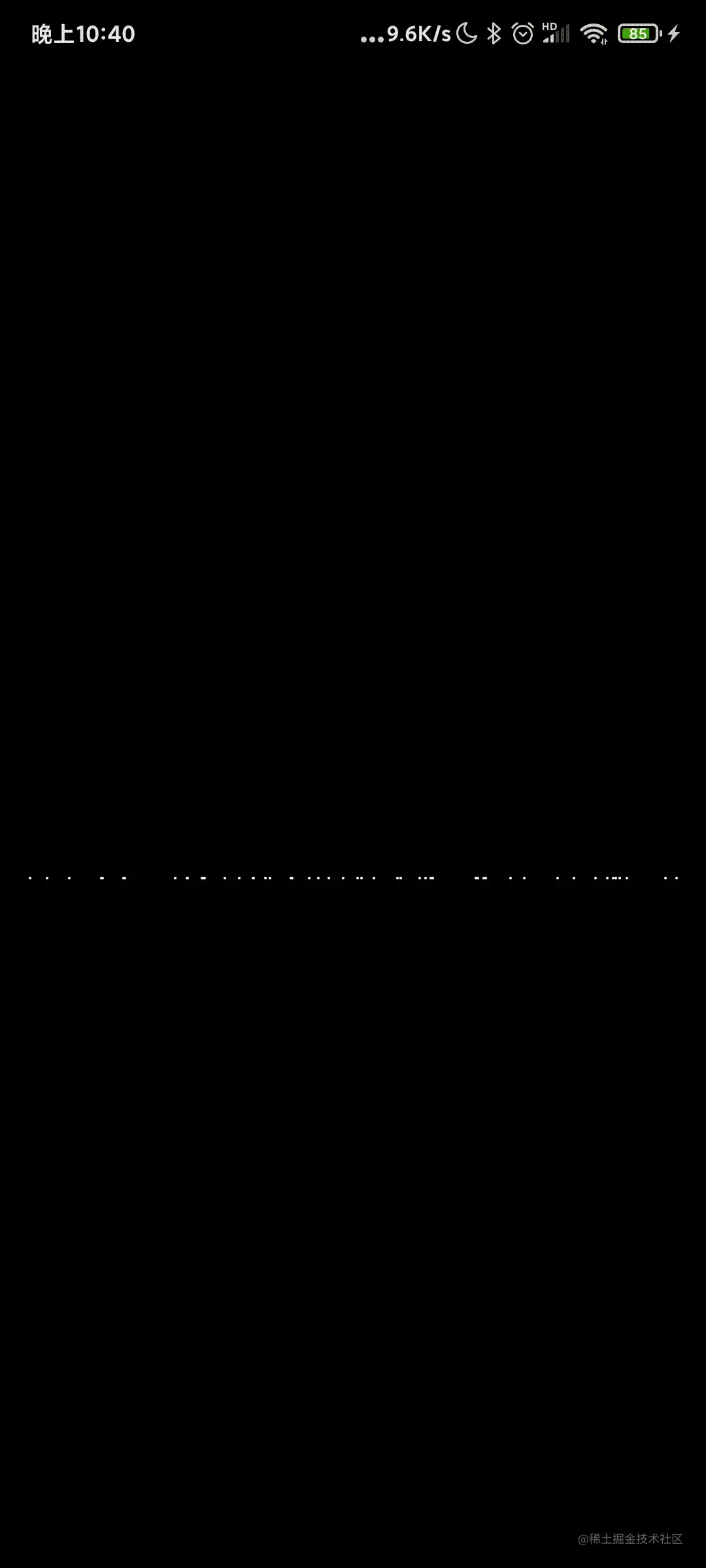emmmm看起来有点雏形了，不过效果图里的粒子速度似乎是随机的，咱们这里是同步的呀。

override fun onSizeChanged(w: Int, h: Int, oldw: Int, oldh: Int) {
super.onSizeChanged(w, h, oldw, oldh)
centerX = (w / 2).toFloat()
centerY = (h / 2).toFloat()
val random = Random()
var nextX = 0
var speed=0 //定义一个速度
for (i in 0..50) {
nextX = random.nextInt((centerX * 2).toInt())
speed= random.nextInt(10)+5 //速度从5-15不等
Particle(nextX.toFloat(), centerY, 2f, speed.toFloat(), 100)
)
}
animator.start()
}


#### 修改粒子的定义

class Particle(
var x:Float,//X坐标
var y:Float,//Y坐标
var speed:Float,//速度
var alpha: Int, //透明度
var maxOffset:Float=300f//最大移动距离
)


#### 粒子运动距离判定

private fun updateParticle(value: Float) {
particleList.forEach {
if(it.y - centerY >it.maxOffset){
it.y=centerY //重新设置Y值
it.x = random.nextInt((centerX * 2).toInt()).toFloat() //随机设置X值
it.speed= (random.nextInt(10)+5).toFloat() //随机设置速度
}
it.y += it.speed
}
}


emmm似乎还不错的样子？不过人家的粒子看起来很多呀，没关系，我们这里设置成300个粒子再试试？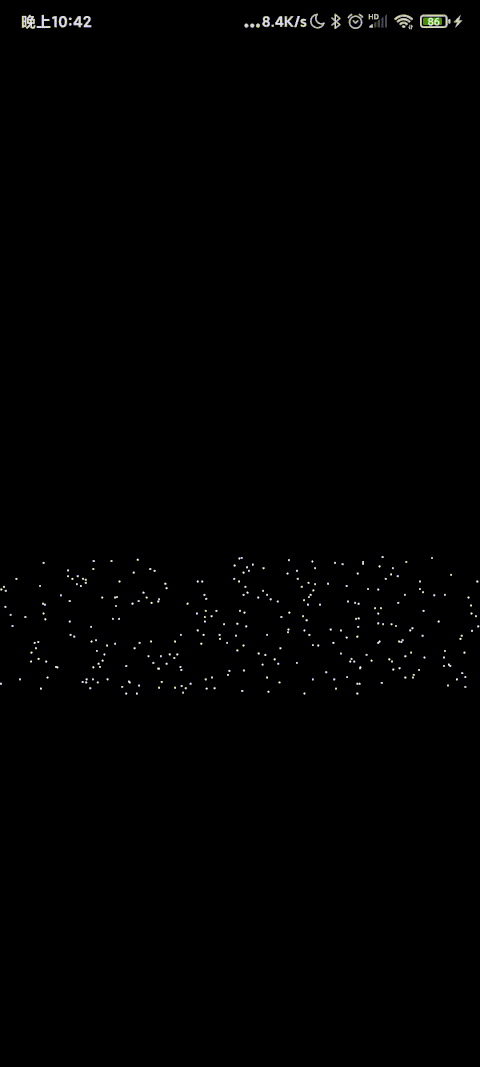#### 粒子移动透明

private fun updateParticle(value: Float) {
particleList.forEach {
...
//设置粒子的透明度
it.alpha= ((1f - (it.y-centerY) / it.maxOffset)  * 225f).toInt()
...
}
}
override fun onDraw(canvas: Canvas) {
...
var time = measureTimeMillis {
particleList.forEach {
//设置画笔的透明度
paint.alpha=it.alpha
}
}
...
}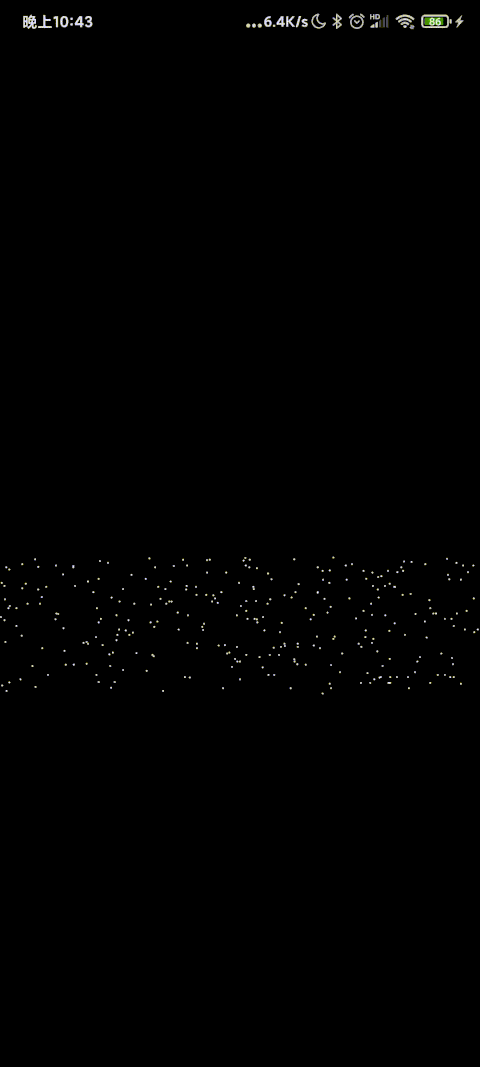override fun onSizeChanged(w: Int, h: Int, oldw: Int, oldh: Int) {
super.onSizeChanged(w, h, oldw, oldh)
...
var nextY=0f
for (i in 0..500) {
...
//初始化Y值，这里是以起始点作为最低值，最大距离作为最大值
nextY= random.nextInt(400)+centerY
speed= random.nextInt(10)+5
Particle(nextX.toFloat(), nextY, 2f, speed.toFloat(), 100)
)
}
animator.start()
}


### 定义圆形

var path = Path()
override fun onSizeChanged(w: Int, h: Int, oldw: Int, oldh: Int) {
...
...
}private val pathMeasure = PathMeasure()//路径，用于测量扩散圆某一处的X,Y值
private var pos = FloatArray(2) //扩散圆上某一点的x,y
private val tan = FloatArray(2)//扩散圆上某一点切线


PathMeasure有个很重要的方法就是getPosTan方法。

boolean getPosTan (float distance, float[] pos, float[] tan)


distance距离 Path 起点的长度取值范围: 0 <= distance <= getLength
pos该点的坐标值当前点在画布上的位置，有两个数值，分别为x，y坐标。
tan该点的正切值当前点在曲线上的方向，使用 Math.atan2(tan, tan) 获取到正切角的弧度值。

#### 圆形添加粒子

override fun onSizeChanged(w: Int, h: Int, oldw: Int, oldh: Int) {
super.onSizeChanged(w, h, oldw, oldh)
centerX = (w / 2).toFloat()
centerY = (h / 2).toFloat()
pathMeasure.setPath(path, false) //添加path
var nextX = 0f
var speed=0
var nextY=0f
for (i in 0..500) {
//按比例测量路径上每一点的值
pathMeasure.getPosTan(i / 500f * pathMeasure.length, pos, tan)
nextX = pos+random.nextInt(6) - 3f //X值随机偏移
nextY=  pos+random.nextInt(6) - 3f//Y值随机偏移
speed= random.nextInt(10)+5
Particle(nextX, nextY, 2f, speed.toFloat(), 100)
)
}
animator.start()
}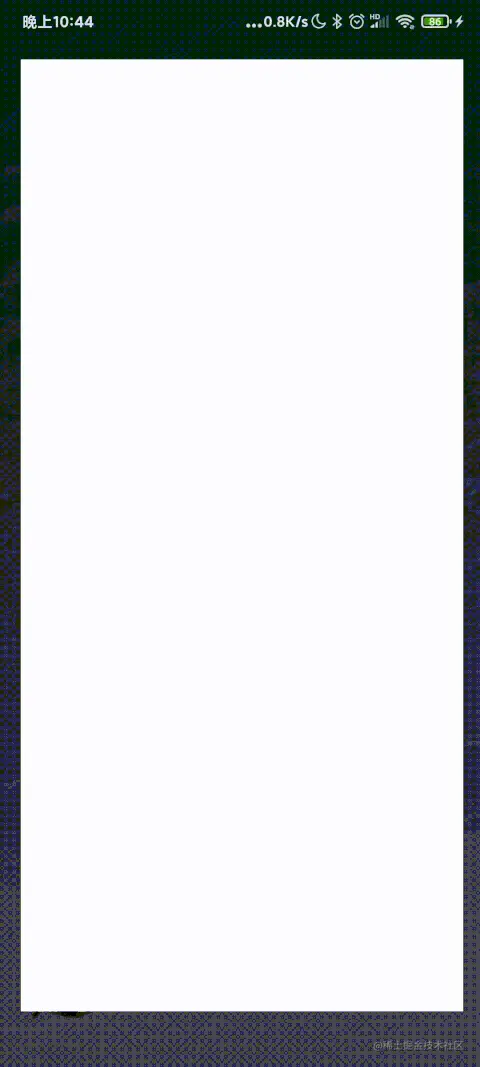### 修改动画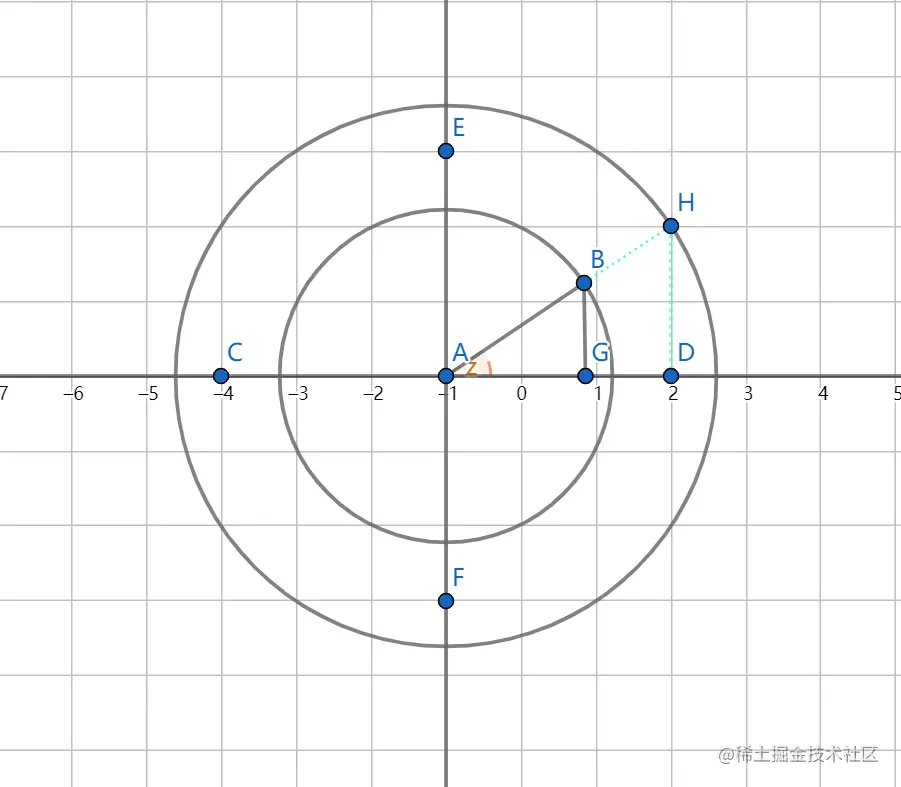AB的值我们是知道的，就是我们一开始画的圆的半径嘛。可是AH是多少呢？

AH=AB +offset

AH知道了，∠Z也知道了，利用三角函数我们可以得到H点的坐标了。设初始半径为R=AB

A点为原点，$cos(∠Z)=AG/AB，sin(∠Z)=BG/AB$

$AD = AH * cos(∠Z) = (AH * AG)/AB=( (R+offset) * AG) / R$

HD

$HD = AH * sin(∠Z) = (AH*BG)/AB = ( (R+offset) * BG) / R$

$AD = ( (R+offset) * (B.X - centerX)) / R$
$HD = ( (R+offset) * (centerY-B.Y) ) / R$

$H.X=AD+centerX=( (R+offset) * (B.X - centerX)) / R + centerX$
$H.Y = centerY - HD = centerY -( (R+offset) * (centerY-B.Y) ) / R$$Z = (B.X -centerX) / R$

$cos∠Z = 0.5$

$H.X= AH * 0.5 +centerX$

$cos∠Z= -0.5$

$H.X= centerX - AH * 0.5$

$H.X = centerX + AH * cos∠Z$

$H.Y = centerY - AH * abs( sin∠Z)$

$H.Y = centerY + AH * abs( sin∠Z)$

class Particle(
...
var offset:Int,//当前移动距离
var angle:Double,//粒子角度
...
)


override fun onSizeChanged(w: Int, h: Int, oldw: Int, oldh: Int) {
...
var angle=0.0
for (i in 0..500) {
...
//反余弦函数可以得到角度值，是弧度
angle=acos(((pos - centerX) / 280f).toDouble())
speed= random.nextInt(10)+5
Particle(nextX, nextY, 2f, speed.toFloat(), 100,0,angle)
)
}
animator.start()
}


private fun updateParticle(value: Float) {
particleList.forEach {particle->
if(particle.offset >particle.maxOffset){
particle.offset=0
particle.speed= (random.nextInt(10)+5).toFloat()
}
particle.alpha= ((1f - particle.offset / particle.maxOffset)  * 225f).toInt()
particle.x = (centerX+ cos(particle.angle) * (280f + particle.offset)).toFloat()
if (particle.y > centerY) {
particle.y = (sin(particle.angle) * (280f + particle.offset) + centerY).toFloat()
} else {
particle.y = (centerY - sin(particle.angle) * (280f + particle.offset)).toFloat()
}
particle.offset += particle.speed.toInt()
}
}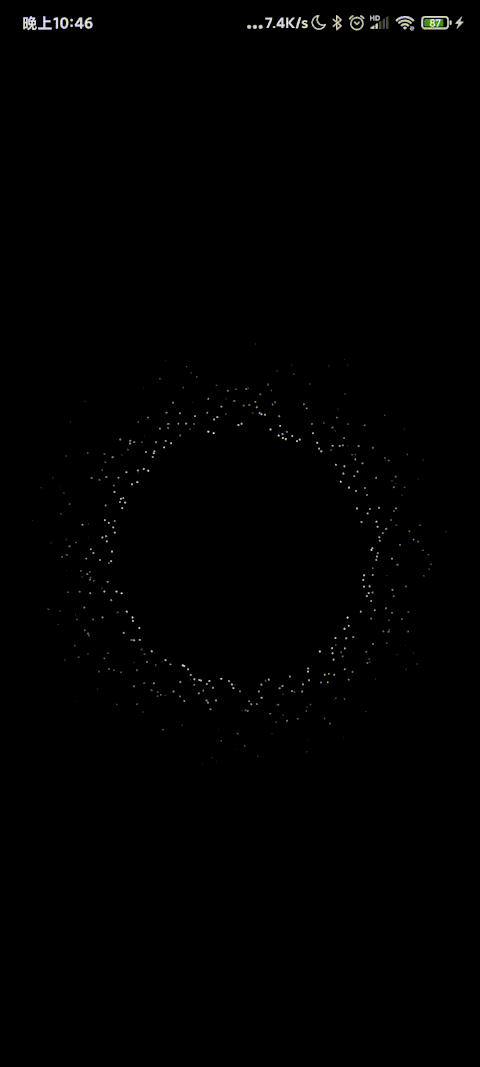override fun onSizeChanged(w: Int, h: Int, oldw: Int, oldh: Int) {
super.onSizeChanged(w, h, oldw, oldh)
centerX = (w / 2).toFloat()
centerY = (h / 2).toFloat()
pathMeasure.setPath(path, false)
var nextX = 0f
var speed=0f
var nextY=0f
var angle=0.0
var offSet=0
var maxOffset=0
for (i in 0..2000) {
pathMeasure.getPosTan(i / 2000f * pathMeasure.length, pos, tan)
nextX = pos+random.nextInt(6) - 3f
nextY=  pos+random.nextInt(6) - 3f
angle=acos(((pos - centerX) / 280f).toDouble())
speed= random.nextInt(2) + 2f
offSet = random.nextInt(200)
maxOffset = random.nextInt(200)
Particle(nextX, nextY, 2f, speed, 100,offSet.toFloat(),angle, maxOffset.toFloat())
)
}
animator.start()
}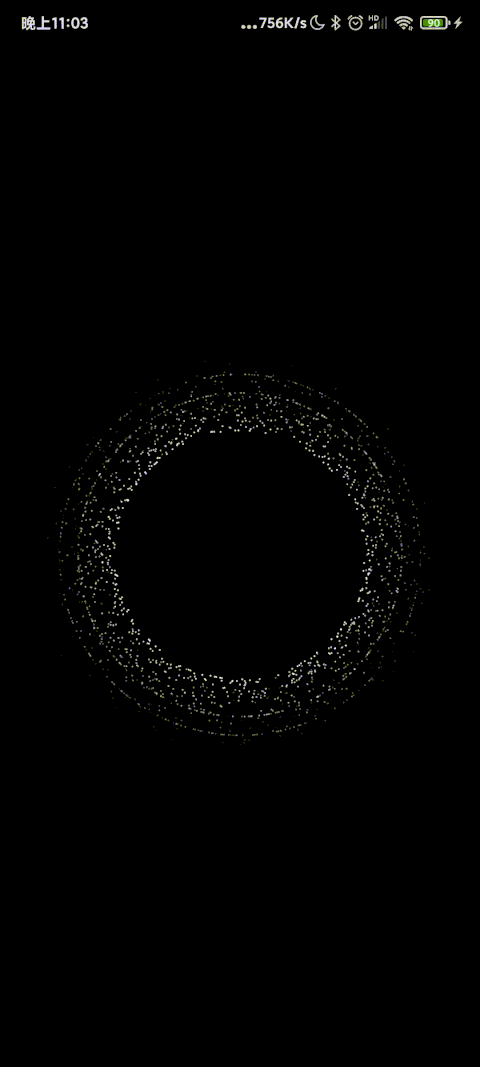## 预告